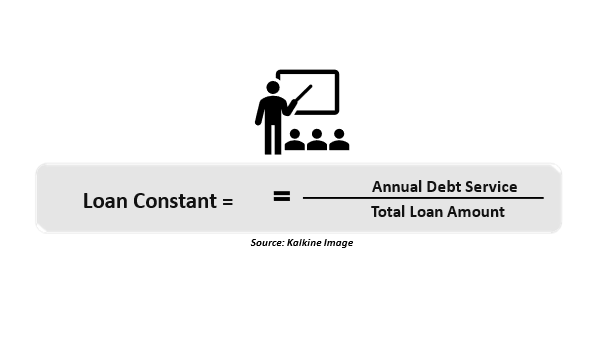# Loan Constant

• Updated on

What is a loan constant?

The loan constant, also known as the mortgage constant, is a percentage that displays the annual debt payment on a loan as a proportion of the entire principal value.

Borrowers could compare the loan constants of several loans before coming to a decision. The borrower picks the loan with the lowest loan constant to reduce the debt service requirements, meaning the borrower will pay less in principal and interest over time.

Summary
• A loan constant is a percentage that measures the annual debt service on a loan to the loan's total principal value.
• A loan constant is estimated using the loan interest rate, principal, and payment length and frequency.
• For estimating mortgage payments, loan constant tables and calculators are used.

How does a loan constant function?

The annual debt payments on a loan are compared to the entire principal value of the loan. Thus, the debt payment on a loan is the total amount of money the borrower will have to pay to cover the repayment of the loan's principal and interest over a set period.

What is a loan constant? | How to calculate loan constant?

In the business and financial worlds, the term "principal" has several connotations. The initial size of a loan or a bond is referred to as principal in the context of borrowing. For instance, if you borrow AU\$80,000, the principal will be AU\$80,000. If you pay down AU\$50,000, the remaining AU\$30,000 is the principal balance.

Furthermore, the amount of interest you will pay on a loan is determined by the principal. For example, if your loan has a principal of AU\$30,000 and a 10% annual interest rate, you will be obliged to pay AU\$3,000 in interest for each year the loan is due.

The loan constant is a percentage that may be determined for any loan. It enables analysts and borrowers to understand better the factors that influence a loan and how much they are paying annually compared to the loan principal.

Moreover, the loan constant is an important indicator used by lenders to assess a property's appropriateness for a commercial or multifamily loan.

For lenders, the loan constant can also be a form of cap rate. The cap rate, also known as the capitalisation rate, is a term used in real estate to describe the rate of return on a property based on its net operating income (NOI). In other terms, the cap rate is a return metric utilised to calculate capital payback or possible return on investment.

To define a property's loan constant, a borrower will need to grasp factors such as the term, amortisation, and interest rate of a loan. Amortisation refers to the entire process of repaying debt in pre-determined, scheduled instalments, including interest and principal. In most circumstances, when a loan is issued, a set of fixed payments is defined at the beginning, and the person who gets the loan is accountable for making all the payments. Therefore, borrowers benefit more from loans with a lower loan constant overall.How to calculate a loan constant?

Calculating the loan constant frequently necessitates a borrower obtaining the various terms linked with the loan agreement. The loan interest rate, total principal, frequency of payments, and length of payment are all included in the terms. Obtaining these loan term components enables the monthly payments to be calculated using a simple present-value payment method. Once the monthly payments have been set, the loan constant can be calculated using the following equation:A mortgage borrower, for example, took out an AU\$100,000 loan. The loan has a fixed interest rate of 7% and a period of 25 years. Using a payments calculator, the borrower would pay approximately AU\$84,000 in annual debt service. Therefore, the loan constant for the borrower would be AU\$84,000 / \$100,000, or 8.4%.

The periodic annual payments are calculated by multiplying the loan constant by the original loan principal. To compare the actual cost of borrowing, the loan constant can be employed.

Loan constants are only applicable for fixed interest rates since variable interest rates have various annual debt service amounts dependent on variable interest. Therefore, if borrowers choose between two loans, they will typically choose the lower loan constant since it will require minor debt servicing.

Before the invention of financial calculators, loan constant tables were frequently utilised in the real estate business because they made calculating monthly mortgage payments very simple. Borrowers can use loan constant tables to get pre-populated information about their loan with a quoted loan constant level.

##### Top ASX Listed Companies

Definition:
We use cookies to ensure that we give you the best experience on our website. If you continue to use this site we will assume that you are happy with it. OK